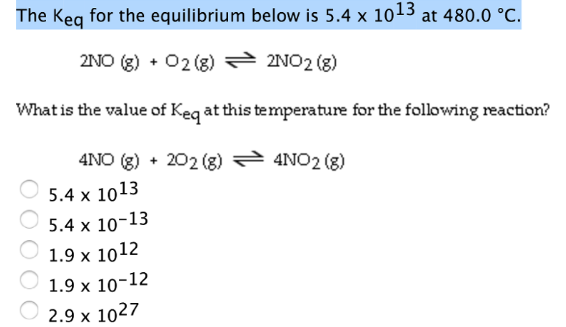# Problem: The Keq for the equillibrium below is 5.4 x 1013 at 480°C.2NO (g) + O2 (g) ⇌ 2NO2 (g)What is the value of Keq at this temperature for the following reaction?4NO (g) + 2O2 (g) ⇌ 4NO2 (g)

###### FREE Expert Solution
87% (185 ratings)###### Problem Details

The Keq for the equillibrium below is 5.4 x 1013 at 480°C.

2NO (g) + O2 (g) ⇌ 2NO2 (g)

What is the value of Keq at this temperature for the following reaction?

4NO (g) + 2O2 (g) ⇌ 4NO2 (g)